# LabVIEW Idea Exchange

View Ideas...
Active
Inactive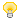Have a LabVIEW Idea?

1. Browse by label or search in the LabVIEW Idea Exchange to see if your idea has previously been submitted. If your idea exists be sure to vote for the idea by giving it kudos to indicate your approval!
2. If your idea has not been submitted click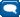Post New Idea to submit a product idea to the LabVIEW Idea Exchange. Be sure to submit a separate post for each idea.
3. Watch as the community gives your idea kudos and adds their input.
4. As NI R&D considers the idea, they will change the idea status.
5. Give kudos to other ideas that you would like to see in a future version of LabVIEW!
Labels
Top Authors
cancel
Showing results for
Did you mean:## Tensorflow API for LabVIEW

Status: New

TensorFlow is an open source machine learning tool originally developed by Google research teams.

Quoting from their API page:

TensorFlow has APIs available in several languages both for constructing and executing a TensorFlow graph. The Python API is at present the most complete and the easiest to use, but the C++ API may offer some performance advantages in graph execution, and supports deployment to small devices such as Android.

Over time, we hope that the TensorFlow community will develop front ends for languages like Go, Java, JavaScript, Lua R, and perhaps others.

Idea Summary: I would love to see LabVIEW among the "perhaps others". 😄

(Disclaimer: I know very little in that particular field of research)LabVIEW Champion. It all comes together inGCentralWhat does "Engineering Redefined" mean??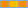Trusted Enthusiast

Is NI member of the TensorFlow community? I read the invitation as one to the open source movement, not necessarily to corporations developing proprietary languages. In particular, I don't suppose that Google is expecting Oracle to develop a Java API... Why leave to NI the responsability to develop a LabVIEW API? Do they have any API to open source standards? I am thinking about HDF5 for instance...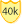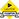Knight of NI

Well, the BLAS pallette is all based on open fortran source. Not sure if that counts.

I am just putting the idea out there. It does not matter if NI or somebody else wants to implement it. 😉LabVIEW Champion. It all comes together inGCentralWhat does "Engineering Redefined" mean??Trusted Enthusiast

Sure. And so do they for most math algorithms they provide, with mixed results. Think of the random number generation algorithms, or the recently uncovered inaccuracies in the Kummer or Parabolic cylinder functions (check my X_Bug_Report tag).

Since we have zero access to the actual implementation, we are basically in the hands of NI engineers for bug fixes. Not a very comfortable place to be...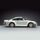Active Participant

I'm starting a project that will need some sort of intelligence. We are looking around for possibilities. TensorFlow may help. Since LabVIEW is not aimed to AI, interfaces to this kind of software may be a nice approach. Kudos!

André Manzolli

Mechanical Engineer
Certified LabVIEW Developer - CLD
LabVIEW Champion
Curitiba - PR - Brazil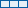Member

Labview has the ability to import a Tensorflow trained model VIA the Vision Development module as of this year!  It has support for both Labview and LabviewRT.  I've used it and I was able to import my model in under and hour using the .pb file.

The API is straight forward:  Open Tensorflow Model, set inputs, read outputs, close.  Further details here:

http://www.ni.com/documentation/en/vision-development-module/latest/ni-vision-algorithms-node-ref/cr...

Feel free to let me know how it goes or what you think.Member

Hello!

I am very happy to see your information.

Can you tell the detailed process? I hope to provide examples and comprehensive examples of each VI in Machine Vision Nodes->Machine Learning Nodes->Deep Learning.
processing. For example, TensorFlow version...TensorFlow code... How to generate files that Labview can load
After generating the files that Labview can load, in the example, the node names are those names in the model? Are the data or pictures those variables...?
Thank you

lijunhuMember

Hi Lijunhu,

Thank you for reaching out.  Here are some more general information.  If your having a specific error, feel free to reach out as well about that.

Current supported Tensorflow version:  Tensorflow 1.4.1  Future releases plan to support later versions.  You may be successful with later versions today, however brand new Tensorflow features won't have support.  Models must be compatible with version 1.4.1.

Tensorflow Model files:  Tensorflow Protocol Buffer (.pb).  Created and maintained by Google(TM).

Supported Platforms:  Windows 64bit;  NI Linux RT 64 bit (many cRIO and vision products are Linux RT 64 bit targets).

Example:  Here is a quick simple example I put together with the importer: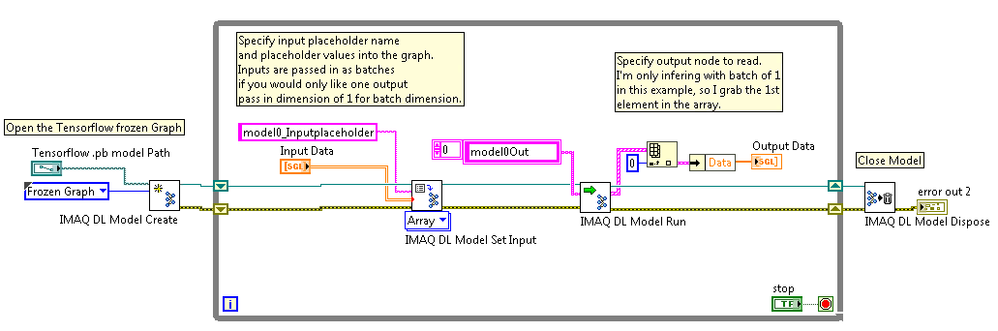Example Python Tensorflow Code to convert your model to a .pb file.  Note that this code is not Labview specific.  It is purely Tensorflow code used to export a tensorflow .pb model file for a third party model importer to open, in our case Labview.  I perform one trick of converting placeholders that I don't care to define in Labview (scaling values, dropout, etc) to Tensorflow graph constants.

```def convert_placeholders_to_constants(input_graph_def,
placeholder_to_value_map):
"""Replaces placeholders in the given tf.GraphDef with constant values.

Args:
input_graph_def: GraphDef object holding the network.
placeholder_to_value_map: A map from the names of placeholder tensors in
`input_graph_def` to constant values.

Returns:
GraphDef containing a simplified version of the original.
"""

print("In convert_placeholders_to_constants")
output_graph_def = tf.GraphDef()

i=0
outputNodes = []
for node in input_graph_def.node:
output_node = tf.NodeDef()
if node.op == "Placeholder" and node.name in placeholder_to_value_map:
output_node.op = "Const"
output_node.name = node.name
dtype = node.attr["dtype"].type
data = np.asarray(placeholder_to_value_map[node.name],
dtype=tf.as_dtype(dtype).as_numpy_dtype)
output_node.attr["dtype"].type = dtype
output_node.attr["value"].CopyFrom(tf.AttrValue(
tensor=tf.contrib.util.make_tensor_proto(data,
dtype=dtype,
shape=data.shape)))
outputNodes.append(output_node)
i += 1
else:
output_node.CopyFrom(node)
outputNodes.append(output_node)

output_graph_def.node.extend(outputNodes)

print("converted ", i, "placeholders to constants")

return output_graph_def

###################################################################
#Main function to save a model
####################################################################
def SaveModel(self):
"""
Saves my Tensorflow Model to a frozen .pb file
"""

################################################################
#For the modelOut0 ouput in my graph,
#convert all associated variables
#(weights, biases, etc) to constants:
################################################################
output_graph_def = tf.graph_util.convert_variables_to_constants(
self.sess, # The session is used to retrieve the weights
tf.get_default_graph().as_graph_def(), # The graph_def is used to retrieve the nodes
["modelOut0"] # The output node names are used to select the usefull nodes
)

################################################################
#Create a dictionary of placeholders-->values
#that I would like to store as constants in the graph
#So that I don't have to pass those as inputs inside of Labview.
################################################################
d =      {}
#set keep_prob to 1 for the dictionary
d.update({ self.keep_prob.op.name: 1})
d.update({self.minLossPlaceholder.op.name: self.minLoss})
d.update({self.lossRangePlaceholder.op.name: self.lossRange})

#Tensorflow doesn't have a function for this.
#I found this function online and copied it into my code
#which converts the placeholders that I don't want to specify in labview to constants.
output_graph_def = convert_placeholders_to_constants(output_graph_def, d)

# Finally we serialize and dump the output graph to the filesystem
with tf.gfile.GFile("tensorflowGraphDefinition.pb", "wb") as myFile:
myFile.write(output_graph_def.SerializeToString())
print("%d ops in the final graph." % len(output_graph_def.node))```

Common Labview Errors:

1. Error -1074395541: This function is not supported in current platform.

Solution：LabVIEW 64bit must be used.

2. Error -1074395539: This error is reported from Tensorflow.

Solution：It is due to different Tensorflow version between saved models and inference environment. Please check your saved models and           inference environment's Tensorflow versions and ensure they are both version 1.4.Member

Hello!

I am very happy to see your information.

Could you provide more information about tensorflow? For example,how to train the network to generate PB files,and then how to call PB file in labview? I have tried too many times according to the help document in NI,but the data identified is wrong.

thank you

ZhankuiHuoMember

Hi ZhankuiHuo,

Tensorflow is Google's machine learning toolkit.  https://www.tensorflow.org/tutorials has information on getting started with Tensorflow.  It is a supported API by Google, in which they are updating with new machine learning concepts from the community.

In order to use your neural network, convolutional nueral network, or other machine learning network in Labview you perform these high level steps.

In tensorflow:

Use Tensorflow to train your machine learning model, performing tasks such as defining neural network architecture, feeding it input data, feeding it expected output data, and performing epochs to have your model learn the connection between input data and output data.

Write a function like the SaveModel function above (comment on 11-05-2018 08:36 AM) to save the trained network into a .PB file (file defining mathematical operations to map your input data to output data).

Or, find an already trained tensorflow model from someone in the community.

In Labview:

I'd love to hear feedback or specific questions you may have about your application or errors seen to further assist.  Right now it does require much learning about tensorflow to use.Member

Hi

I used win7 64 bit , configuration of the tensorflow framework for 1.4.0 and python version of 3.5.2.  in Spyder run *.py file to generate .PB file.

In order to verify whether the .PB file is correct, I also conducted a test in Spyder ,and finally passed the test smoothly.

In  Labview,

I use the .PB file generated by Tensorflow(to judge whether the picture is a dog or a cat) to make picture prediction.When running the same VI, two situations will occur. The first is that the system will not give an error, and the "Data" value in the "Output Data Array" is "NaN". In the second case ,the system does not report an error, and the "Data" value in the "Output Data Array" is a normal floating-point number, but the result is the same regardless of the test image. Pictures are as follows: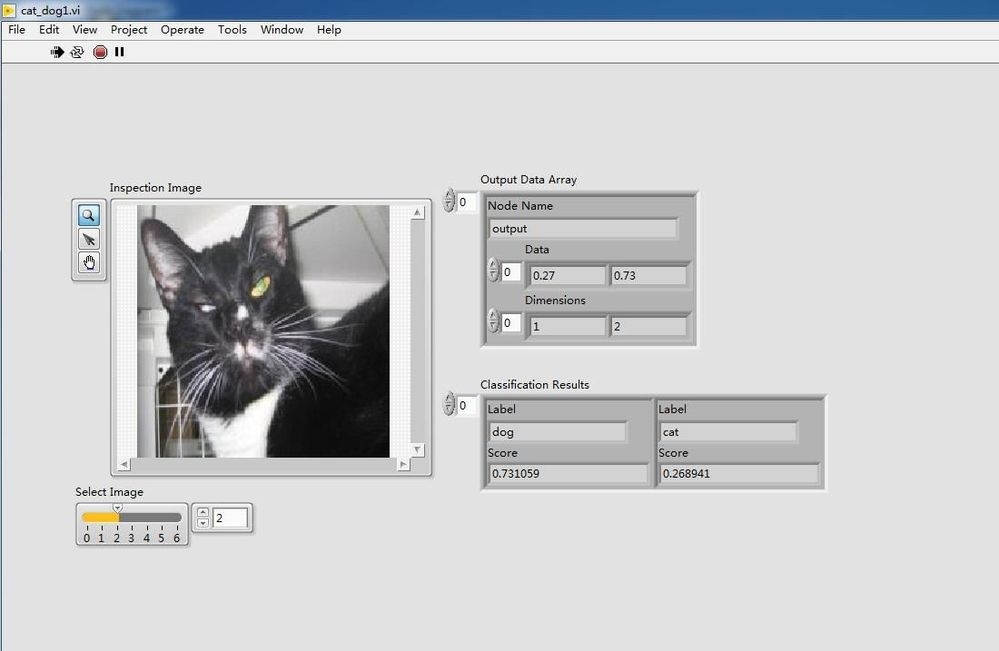tensorflow code：

```######################
######################
import tensorflow as tf
import numpy as np
import os
def get_all_files(file_path, is_random=True):
"""
获取图片路径及其标签
:param file_path: a sting, 图片所在目录
:param is_random: True or False, 是否乱序
:return:
"""
image_list = []
label_list = []

cat_count = 0
dog_count = 0
for item in os.listdir(file_path):
item_path = file_path + '\\' + item
item_label = item.split('.')  # 文件名形如  cat.0.jpg,只需要取第一个

if os.path.isfile(item_path):
image_list.append(item_path)
else:
raise ValueError('文件夹中有非文件项.')

if item_label == 'cat':  # 猫标记为'0'
label_list.append(0)
cat_count += 1
else:  # 狗标记为'1'
label_list.append(1)
dog_count += 1
print('数据集中有%d只猫,%d只狗.' % (cat_count, dog_count))

image_list = np.asarray(image_list)
label_list = np.asarray(label_list)
# 乱序文件
if is_random:
rnd_index = np.arange(len(image_list))
np.random.shuffle(rnd_index)
image_list = image_list[rnd_index]
#print(image_list)
label_list = label_list[rnd_index]
#print(label_list)
return image_list, label_list

def get_batch(train_list, image_size, batch_size, capacity, is_random=True):
"""
获取训练批次
:param train_list: 2-D list, [image_list, label_list]
:param image_size: a int, 训练图像大小
:param batch_size: a int, 每个批次包含的样本数量
:param capacity: a int, 队列容量
:param is_random: True or False, 是否乱序
:return:
"""
#tf.train.slice_input_producer是一个tensor生成器，作用是按照设定，每次从一个tensor列表中按顺序或者随机抽取出一个tensor放入文件名队列。
#如果shuffle=True，生成的样本顺序就被打乱了，在批处理的时候不需要再次打乱样本，使用 tf.train.batch函数就可以了;
#如果shuffle=False,就需要在批处理时候使用 tf.train.shuffle_batch函数打乱样本
intput_queue = tf.train.slice_input_producer(train_list, shuffle=False)
#print(intput_queue)

# 从路径中读取图片
image_train = tf.image.decode_jpeg(image_train, channels=3)  # 这里是jpg格式
image_train = tf.image.resize_images(image_train, [image_size, image_size])
image_train = tf.cast(image_train, tf.float32) / 255.  # 转换数据类型并归一化

# 图片标签
label_train = intput_queue

# 获取批次
if is_random:
image_train_batch, label_train_batch = tf.train.shuffle_batch([image_train, label_train],
batch_size=batch_size,
capacity=capacity,
min_after_dequeue=100,
else:
image_train_batch, label_train_batch = tf.train.batch([image_train, label_train],
batch_size=1,
capacity=capacity,
return image_train_batch, label_train_batch

if __name__ == '__main__':
import matplotlib.pyplot as plt

# 测试图片读取
image_dir = 'data\\train'
train_list = get_all_files(image_dir, True)
image_train_batch, label_train_batch = get_batch(train_list, 256, 1, 200, False)
sess = tf.Session()
coord = tf.train.Coordinator()

try:
for step in range(10):
if coord.should_stop():
break

image_batch, label_batch = sess.run([image_train_batch, label_train_batch])
if label_batch == 0:
label = 'Cat'
else:
label = 'Dog'
plt.imshow(image_batch), plt.title(label)
plt.show()
except tf.errors.OutOfRangeError:
print('Done.')
finally:
coord.request_stop()
sess.close()

```
```#########################
model.py
#########################
import tensorflow as tf
import tensorflow.contrib.layers as layers

def inference(images, n_classes):
# conv1, shape = [kernel_size, kernel_size, channels, kernel_numbers]
with tf.variable_scope("conv1") as scope:
weights = tf.get_variable("weights",
shape=[3, 3, 3, 16],
dtype=tf.float32,
initializer=tf.truncated_normal_initializer(stddev=0.1, dtype=tf.float32))
biases = tf.get_variable("biases",
shape=,
dtype=tf.float32,
initializer=tf.constant_initializer(0.1))
conv = tf.nn.conv2d(images, weights, strides=[1, 1, 1, 1], padding="SAME")
conv1 = tf.nn.relu(pre_activation, name="conv1")

# pool1 && norm1
with tf.variable_scope("pooling1_lrn") as scope:
pool1 = tf.nn.max_pool(conv1, ksize=[1, 3, 3, 1], strides=[1, 2, 2, 1],
norm1 = tf.nn.lrn(pool1, depth_radius=4, bias=1.0, alpha=0.001/9.0,
beta=0.75, name='norm1')

# conv2
with tf.variable_scope("conv2") as scope:
weights = tf.get_variable("weights",
shape=[3, 3, 16, 16],
dtype=tf.float32,
initializer=tf.truncated_normal_initializer(stddev=0.1, dtype=tf.float32))
biases = tf.get_variable("biases",
shape=,
dtype=tf.float32,
initializer=tf.constant_initializer(0.1))
conv = tf.nn.conv2d(norm1, weights, strides=[1, 1, 1, 1], padding="SAME")
conv2 = tf.nn.relu(pre_activation, name="conv2")

# pool2 && norm2
with tf.variable_scope("pooling2_lrn") as scope:
pool2 = tf.nn.max_pool(conv2, ksize=[1, 3, 3, 1], strides=[1, 2, 2, 1],
norm2 = tf.nn.lrn(pool2, depth_radius=4, bias=1.0, alpha=0.001/9.0,
beta=0.75, name='norm2')

# full-connect1
with tf.variable_scope("fc1") as scope:
reshape = layers.flatten(norm2)
dim = reshape.get_shape().value
weights = tf.get_variable("weights",
shape=[dim, 128],
dtype=tf.float32,
initializer=tf.truncated_normal_initializer(stddev=0.005, dtype=tf.float32))
biases = tf.get_variable("biases",
shape=,
dtype=tf.float32,
initializer=tf.constant_initializer(0.1))
fc1 = tf.nn.relu(tf.matmul(reshape, weights) + biases, name="fc1")

# full_connect2
with tf.variable_scope("fc2") as scope:
weights = tf.get_variable("weights",
shape=[128, 128],
dtype=tf.float32,
initializer=tf.truncated_normal_initializer(stddev=0.005, dtype=tf.float32))
biases = tf.get_variable("biases",
shape=,
dtype=tf.float32,
initializer=tf.constant_initializer(0.1))
fc2 = tf.nn.relu(tf.matmul(fc1, weights) + biases, name="fc2")

# softmax
with tf.variable_scope("softmax_linear") as scope:
weights = tf.get_variable("weights",
shape=[128, n_classes],
dtype=tf.float32,
initializer=tf.truncated_normal_initializer(stddev=0.005, dtype=tf.float32))
biases = tf.get_variable("biases",
shape=[n_classes],
dtype=tf.float32,
initializer=tf.constant_initializer(0.1))
softmax_linear = tf.add(tf.matmul(fc2, weights), biases, name="softmax_linear")
out = tf.nn.softmax(softmax_linear, name = "output")

return out

def losses(logits, labels):
with tf.variable_scope('loss'):
cross_entropy = tf.nn.sparse_softmax_cross_entropy_with_logits(logits=logits,
labels=labels)
loss = tf.reduce_mean(cross_entropy)
return loss

def evaluation(logits, labels):
with tf.variable_scope("accuracy"):
correct = tf.nn.in_top_k(logits, labels, 1)
correct = tf.cast(correct, tf.float16)
accuracy = tf.reduce_mean(correct)
return accuracy
```
```###########################
train.py
###########################
import time
from model import *
import matplotlib.pyplot as plt
from tensorflow.python.framework import graph_util
from tensorflow.python.framework.graph_util import convert_variables_to_constants

# 训练模型
def training():
N_CLASSES = 2
IMG_SIZE = 208
BATCH_SIZE = 8
CAPACITY = 200
MAX_STEP = 12000
LEARNING_RATE = 1e-4

# 测试图片读取
image_dir = 'data\\train'
logs_dir = 'logs_2'     # 检查点保存路径

sess = tf.Session()

train_list = get_all_files(image_dir, True)
image_train_batch, label_train_batch = get_batch(train_list, IMG_SIZE, BATCH_SIZE, CAPACITY, True)
x_image = tf.placeholder(tf.float32, shape=[BATCH_SIZE, IMG_SIZE, IMG_SIZE, 3], name= "input")

train_logits = inference(x_image, N_CLASSES)

train_loss = losses(train_logits, label_train_batch)

train_acc = evaluation(train_logits, label_train_batch)

var_list = tf.trainable_variables()
paras_count = tf.reduce_sum([tf.reduce_prod(v.shape) for v in var_list])
print('参数数目:%d' % sess.run(paras_count), end='\n\n')

saver = tf.train.Saver()

sess.run(tf.global_variables_initializer())

coord = tf.train.Coordinator()

s_t = time.time()
try:
for step in range(MAX_STEP):
if coord.should_stop():
break
tra_image, tra_prediction = sess.run([image_train_batch, label_train_batch])
_, loss, acc = sess.run([train_op, train_loss, train_acc], feed_dict = {x_image: tra_image})
#_, loss, acc = sess.run([train_op, train_loss, train_acc])

if step % 100 == 0:  # 实时记录训练过程并显示
runtime = time.time() - s_t
print('Step: %6d, loss: %.8f, accuracy: %.2f%%, time:%.2fs, time left: %.2fhours'
% (step, loss, acc * 100, runtime, (MAX_STEP - step) * runtime / 360000))
s_t = time.time()

if step % 1000 == 0 or step == MAX_STEP - 1:  # 保存检查点
checkpoint_path = os.path.join(logs_dir, 'model.ckpt')
saver.save(sess, checkpoint_path, global_step=step)

except tf.errors.OutOfRangeError:
print('Done.')
finally:
coord.request_stop()

sess.close()

# 测试检查点
def eval():
N_CLASSES = 2
IMG_SIZE = 208
BATCH_SIZE = 1
CAPACITY = 200
MAX_STEP = 100

test_dir = 'C:\\Users\\vecow\\tensorflow_study_01\\Cats_vs_Dogs-master_wangluoban\\Cats_vs_Dogs-master22222222222\\Cats_vs_Dogs-master\\data\\test'
logs_dir = 'logs_1'     # 检查点目录

sess = tf.Session()
#    sess = tf.Session(config=tf.ConfigProto(log_device_placement=True))

#    config = tf.ConfigProto(allow_soft_placement=True,log_device_placement=True)
#    sess=tf.Session(config=config)

train_list = get_all_files(test_dir, is_random=True)
#print(train_list)
#print(train_list)
image_train_batch, label_train_batch = get_batch(train_list, IMG_SIZE, BATCH_SIZE, CAPACITY, True)

x_image = tf.placeholder(tf.float32, shape=[BATCH_SIZE, IMG_SIZE, IMG_SIZE, 3], name= "input")
train_logits = inference(x_image, N_CLASSES)

y_output = tf.nn.softmax(train_logits, name = "output")  # 用softmax转化为百分比数值

# 载入检查点
saver = tf.train.Saver()
print('\n载入检查点...')
ckpt = tf.train.get_checkpoint_state(logs_dir)
if ckpt and ckpt.model_checkpoint_path:
global_step = ckpt.model_checkpoint_path.split('/')[-1].split('-')[-1]
#print(global_step)
saver.restore(sess, ckpt.model_checkpoint_path)
print('载入成功，global_step = %s\n' % global_step)
else:
print('没有找到检查点')

coord = tf.train.Coordinator()

try:
for step in range(MAX_STEP):
if coord.should_stop():
break

x_in = sess.run(image_train_batch)
#image, prediction = sess.run([image_train_batch, train_logits])
image, prediction = sess.run([x_image, train_logits], feed_dict ={x_image: x_in})
#tra_prediction = sess.run(train_logits,feed_dict = {x_image: image_train_batch})
max_index = np.argmax(prediction)
if max_index == 0:
label = '%.2f%% is a cat.' % (prediction * 100)
else:
label = '%.2f%% is a dog.' % (prediction * 100)
#y_label = label

plt.imshow(image)
plt.title(label)
plt.show()

except tf.errors.OutOfRangeError:
print('Done.')
finally:
coord.request_stop()

constant_graph = graph_util.convert_variables_to_constants(sess, sess.graph_def,["output"])
with tf.gfile.FastGFile(pb_file_path, mode='wb') as f:
f.write(constant_graph.SerializeToString())